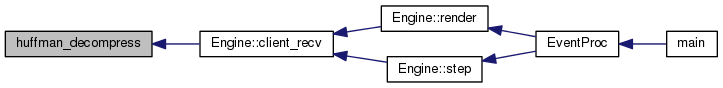altEngine
huffman.h File Reference
This graph shows which files directly or indirectly include this file: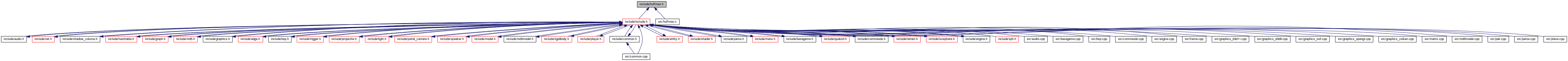Go to the source code of this file.

## Macros

#define HUFFHEAP_SIZE   ((sizeof(double) * 257) + (((sizeof(void *) * 4) + sizeof(double) + sizeof(unsigned long)) * (257 * 3)) + ((sizeof(unsigned long) + sizeof(unsigned long)) * 257))

## Functions

unsigned long huffman_compress (const unsigned char *in, unsigned long inlen, unsigned char *out, unsigned long outlen, void *huffheap)

unsigned long huffman_decompress (const unsigned char *in, unsigned long inlen, unsigned char *out, unsigned long outlen, void *huffheap)

## Macro Definition Documentation

 #define HUFFHEAP_SIZE   ((sizeof(double) * 257) + (((sizeof(void *) * 4) + sizeof(double) + sizeof(unsigned long)) * (257 * 3)) + ((sizeof(unsigned long) + sizeof(unsigned long)) * 257))

Required size of huffheap parameter to compress and decompress

Note: if you change any of the data types in the _huffman_node or _huffman_encode_table structs in huffman.c, this also must be changed.

## Function Documentation

 unsigned long huffman_compress ( const unsigned char * in, unsigned long inlen, unsigned char * out, unsigned long outlen, void * huffheap )

Huffman encode a block of data

Parameters
 in Input data inlen Input data length out Output buffer outlen Output buffer length huffheap Heap memory to use for compression (must be HUFFHEAP_SIZE in size)
Returns
Size of encoded result or 0 on out buffer overrun

Here is the call graph for this function: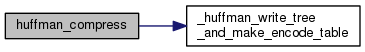Here is the caller graph for this function: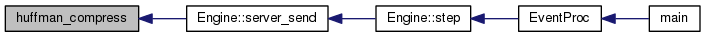unsigned long huffman_decompress ( const unsigned char * in, unsigned long inlen, unsigned char * out, unsigned long outlen, void * huffheap )

Huffman decode a block of data

Parameters
 in Input data inlen Length of input data out Output buffer outlen Length of output buffer huffheap Heap memory to use for decompression (must be HUFFHEAP_SIZE in size)
Returns
Size of decoded result or 0 on out buffer overrun or corrupt input data

Here is the call graph for this function: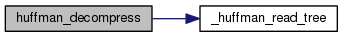Here is the caller graph for this function: